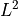# sfepy.discrete.projections module¶

Construct projections between FE spaces.

`sfepy.discrete.projections.``create_mass_matrix`(field)[source]

Create scalar mass matrix corresponding to the given field.

Returns
mtxcsr_matrix

The mass matrix in CSR format.

`sfepy.discrete.projections.``make_h1_projection_data`(target, eval_data)[source]

Project scalar data given by a material-like eval_data() function to a scalar target field variable using thedot product.

`sfepy.discrete.projections.``make_l2_projection`(target, source, ls=None, nls_options=None)[source]

Project a scalar source field variable to a scalar target field variable using thedot product.

`sfepy.discrete.projections.``make_l2_projection_data`(target, eval_data, order=None, ls=None, nls_options=None)[source]

Project scalar data to a scalar target field variable using thedot product.

Parameters
targetFieldVariable instance

The target variable.

eval_datacallable or array

Either a material-like function eval_data(), or an array of values in quadrature points that has to be reshapable to the shape required by order.

orderint, optional

The quadrature order. If not given, it is set to 2 * target.field.approx_order.

`sfepy.discrete.projections.``project_by_component`(tensor, tensor_qp, component, order, ls=None, nls_options=None)[source]

Wrapper around make_l2_projection_data() for non-scalar fields.